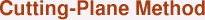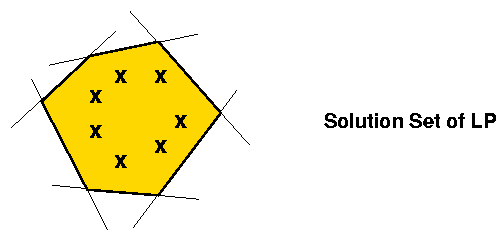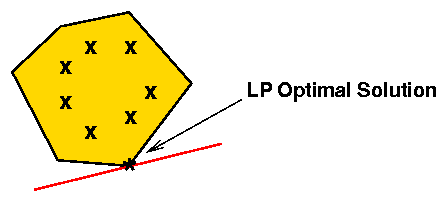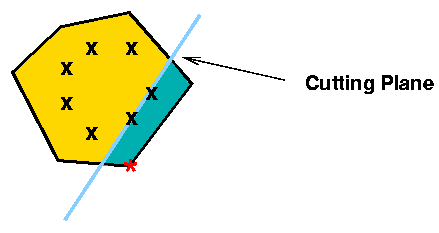Some insight into cutting planes can be gained by looking at a general two-variable model, although this obviously does not apply directly to the TSP.

Let us suppose that all of the constraints of an LP are in <= form (it is always possible to write the constaints in this way).  Then the solution set of the LP is the intersection of a number of half-spaces determined by the constraints.  Such a set is called a polyhedron in general, and with just two variables it is a polgonal region of the plane, as indicated in the following figure.